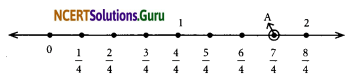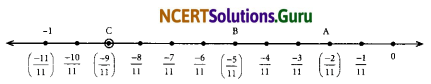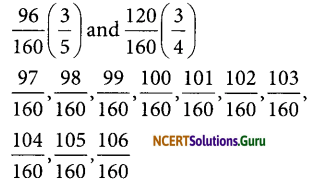# NCERT Solutions for Class 8 Maths Chapter 1 Rational Numbers Ex 1.2

These NCERT Solutions for Class 8 Maths Chapter 1 Rational Numbers Ex 1.2 Questions and Answers are prepared by our highly skilled subject experts.

## NCERT Solutions for Class 8 Maths Chapter 1 Rational Numbers Exercise 1.2

Question 1.
Represent these numbers on the number line:
(i) $$\frac{7}{4}$$
(ii) $$\frac{-5}{6}$$
Solution:
(i) $$\frac{7}{4}$$
We make 7 markings at distance of $$\frac{1}{4}$$ each on the right of 0 and starting from 0, the seventh marking represents $$\frac{7}{4}$$.(ii) $$\frac{-5}{6}$$
We make 5 markings at distance of $$\frac{1}{6}$$ each on the left of ‘0’ and starting from ‘0’, the fifth marking represents $$\frac{-5}{6}$$Question 2.
Represent $$\frac{-2}{11}, \frac{-5}{11}, \frac{-9}{11}$$ on a number line.
Solution:
We make 9 markings at distance of $$\frac{1}{11}$$ each on the left of ‘0’ and starting from ‘0’, the second marking represents $$\frac{-2}{11}$$; the fifth marking represents $$\frac{-5}{11}$$ and the ninth marking represents $$\frac{-9}{11}$$.
The point A represents $$\frac{-2}{11}$$, the point B represents $$\frac{-5}{11}$$ and the point C represents $$\frac{-9}{11}$$.Question 3.
Write five rational numbers which are smaller than 2.
Solution:
There can be infinite rational numbers smaller than 2.
Five rational numbers are 0, -1, $$\frac{1}{2}$$, $$\frac{-1}{2}$$, 1Question 4.
Find ten rational numbers between $$\frac{-2}{5}$$ and $$\frac{1}{2}$$
Solution:
Convert $$\frac{-2}{5}$$ and $$\frac{1}{2}$$ with the same denominators.
$$\frac{1}{2}=\frac{1 \times 5}{2 \times 5}=\frac{5}{10}$$
$$\frac{-2}{5}=\frac{2 \times 2}{5 \times 2}=\frac{-4}{10}$$
To get ten rational numbers, multiply both numerator and denominator by 2
$$\frac{5}{10}=\frac{5 \times 2}{10 \times 2}=\frac{10}{20}$$
$$\frac{-4}{10}=\frac{-4 \times 2}{10 \times 2}=\frac{-8}{20}$$
The rational numbers between $$\frac{10}{20}$$ and $$\frac{-8}{20}$$ are
$$\frac{9}{20}, \frac{8}{20}, \frac{7}{20}, \frac{6}{20}, \frac{5}{20}, \frac{4}{20}, \frac{3}{20}, \ldots \frac{-6}{20}, \frac{-7}{20}$$
We can take any 10 of them.

Question 5.
Find five rational numbers between
(i) $$\frac{2}{3}$$ and $$\frac{4}{5}$$
(ii) $$\frac{-3}{2}$$ and $$\frac{5}{3}$$
(iii) $$\frac{1}{4}$$ and $$\frac{1}{2}$$
Solution:
Convert $$\frac{2}{3}$$ and $$\frac{4}{5}$$ with the same denominators
$$\frac{2}{3}=\frac{2 \times 5}{3 \times 5}=\frac{10}{15}$$
$$\frac{4}{5}=\frac{4 \times 3}{5 \times 3}=\frac{12}{15}$$
The difference between the numerators should be more than 5
$$\frac{10}{15}=\frac{10 \times 4}{15 \times 4}=\frac{40}{60}$$
$$\frac{12}{15}=\frac{12 \times 4}{15 \times 4}=\frac{48}{60}$$
Five rational numbers between $$\frac{40}{60}$$ and $$\frac{48}{60}$$ are
$$\frac{41}{60}, \frac{42}{60}, \frac{43}{60}, \frac{44}{60}, \frac{45}{60}$$

(ii) Convert $$\frac{-3}{2}$$ and $$\frac{5}{3}$$ with the same denominators.
$$\frac{-3}{2}=\frac{-3 \times 3}{2 \times 3}=\frac{-9}{6}$$
$$\frac{5}{3}=\frac{5 \times 2}{3 \times 2}=\frac{10}{6}$$
Five rational numbers between $$\frac{-9}{6}$$ and $$\frac{10}{6}$$ are $$\frac{9}{6}, \frac{8}{6}, \frac{7}{6}, 0, \frac{-7}{6}$$

(iii) Convert $$\frac{1}{4}$$ and $$\frac{1}{2}$$ with the same denominators
$$\frac{1}{4}=\frac{1}{4}$$
$$\frac{1}{2}=\frac{1 \times 2}{2 \times 2}=\frac{2}{4}$$
The difference between the numerators should be more than 5
$$\frac{1}{4}=\frac{1 \times 8}{4 \times 8}=\frac{8}{32}$$
$$\frac{2}{4}=\frac{2 \times 8}{4 \times 8}=\frac{16}{32}$$
Five rational numbers between $$\frac{8}{32}$$ and $$\frac{16}{32}$$ are $$\frac{9}{32}, \frac{10}{32}, \frac{11}{32}, \frac{12}{32}, \frac{13}{32}$$Question 6.
Write five rational numbers greater than -2.
Solution:
There are infinite rational numbers greater than -2.
The numbers are $$\frac{-3}{2},-1, \frac{-1}{2}, 0, \frac{1}{2}$$

Question 7.
Find ten rational numbers between $$\frac{3}{5}$$ and $$\frac{3}{4}$$.
Solution:
Convert $$\frac{3}{5}$$ and $$\frac{3}{4}$$ with the same denominators
$$\frac{3}{5}=\frac{3 \times 4}{5 \times 4}=\frac{12}{20}$$
$$\frac{3}{4}=\frac{3 \times 5}{4 \times 5}=\frac{15}{20}$$
The difference between the numerator should be more than 10.
$$\frac{12}{20}=\frac{12 \times 8}{20 \times 8}=\frac{96}{160}$$
$$\frac{15}{20}=\frac{15 \times 8}{20 \times 8}=\frac{120}{160}$$
Thus, ten rational numbers betweenerror: Content is protected !!